Level Specification

The concept of level specification is explained in The Mathematica Book  (Sections 2.1.7, and A.3.6), and is explained further here.  The "level" of an expression is  fundamental to an understanding of level specification.  Level 1 of an  expression is all subexpressions that can be accessed by giving Part a single  integer position (e.g.   expr[]).  Level 2 of an expression is all  subexpressions that can be accessed by giving Part two integers to specify  the position (e.g.    expr[[2,3]]).  The parts of an expression at level (n)  can be accessed by giving Part a sequence of (n) integers.  Level 0 of an  expression is the expression itself.  As indicated by the usage message for  Level (below) the second argument of Level is a level specification.  In the  cells that follow Level[demo,_] will be used to explore the different  variations of level specification.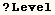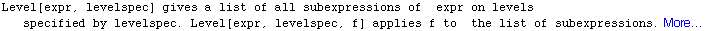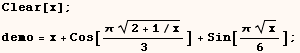The functions (Apply, Cases, Count, DeleteCases, FreeQ, Level, Map,  MapIndexed, MemberQ, Position, Replace, Scan) can  use level specification  with the same conventions described below.  Several examples of using level  specification are given in the discussion of Map in addition to those in this discussion of Level.  Each of these commands  also have the option Heads.  The Heads option can be True or False and  controls whether the command should look at the heads of subexpressions.

A positive integer for a level specification

When (levelspec) in Level[expr,levelspec] is an integer (n) a list of all
subexpressions of (expr) at all levels from 1 through (n) is returned.  In
the cell below Level is used to get a list of all subexpressions of demo at
level 1.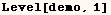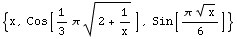In the next line Level[demo, 2] returns a list of all subexpressions at
levels 1 through 2.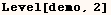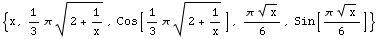A list of one positive integer for a level specification

If the level specification given to Level is a list containing one positive
integer, then only subexpressions at the level in the list are returned.  In
the next cell Level gives a list of all subexpressions at level 2.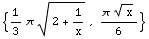A level specification deeper than the expression can be given.  When Level
gets such a level specification it returns an empty list as in the following
line.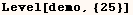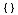A list of two positive integers as a level specification

If Level is given a list of two integers {m,n} as a level specification, then
we get a list of all subexpressions at level (m) at level (n) and all
subexpressions at levels between (m) and (n).  The next line returns a list
of all subexpressions at levels 2, 3, and 4.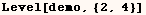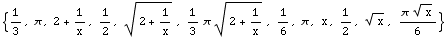The next line gives a list of all subexpressions at levels 0, 1, and 2.  The
subexpression at level 0 is the expression itself.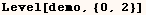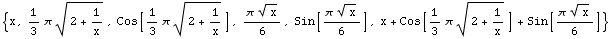Negative integers in level specifications

To understand negative levels you have to first understand the Mathematica
meaning of depth.  The depth of an expression is said to be one greater than
the maximum number of indices needed to access any part of an expression.

A list of one negative integer for a level specification

A level specification {-n} (where -n is a negative integer) is all
subexpressions that have depth (n).  Atoms have depth 1, so the level
specification {-1} in the next line causes Level to return a list of all the
atoms in (demo2).

Note:  Rational numbers have a Numerator and Denominator.  However the
numerator and denominator of rational numbers can't  be accessed using Part
so they are regarded as atoms and have a depth of 1.  Complex numbers are
also regarded as atoms although none were included in (demo).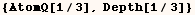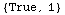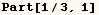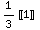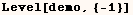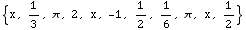In the next line the level specification is {-4}, and Level returns a list of
all subexpressions of demo with depth equal to 4.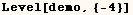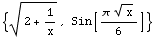As with positive integer level specifications Level may return an empty list
if the given expression has no subexpressions sufficiently deep.  The next
line returns an empty list because neither demo or any of it's subexpressions
have a depth of 25.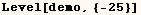A negative integer as a level specification

If Level is given a negative integer as a level specification it returns all
subexpressions from level 1 down to (and including) the negative level
specified.  The next line returns a list of all subexpressions from level 1
down to  those at level -4.  Since the level specification goes down to -4
all sub expressions returned have a depth  of 4 or greater.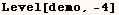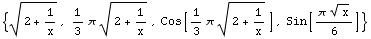If Level is given (-1) as a level specification (as in the next line) we get
a list of all subexpressions from level 1 down to the atoms.  This amounts to
every subexpression, but not the whole expression itself.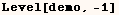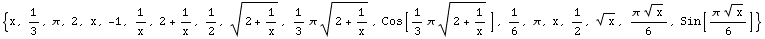In the next line we see level specifications -1 and ∞ are
equivalent.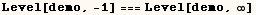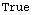A list of integers including one or two negative integers for a level
specification

The next line gives a list of all subexpressions at any level (except level
0) that have a depth of 3 or greater.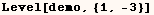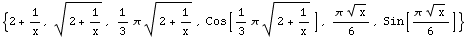Then the next line gives a list of all subexpressions at levels 2 or above
with a depth of 4 or less.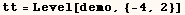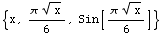In the next two cells level specification {0,-1}  is effectively the same as
level specification {-∞,∞}.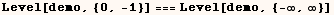In the next line Level is asked to return all subexpressions at levels 3 or
deeper that have a depth of 6 or greater.  Expression demo2 has no
subexpressions meeting this criteria, so an empty list is returned.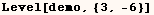The next line gives a list of all subexpression with a depth of 1, 2, or 3.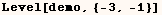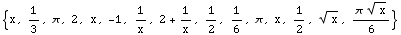Created by Mathematica  (May 16, 2004)

Back to Ted’s Tricks index page